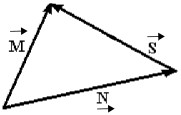# Problem: For the vectors shown in the figure, express vector S→ in terms of vectors M→ and N→.Express your answer in terms of the variables M→ and N→.

###### FREE Expert Solution

In this problem, we'll practice the addition of vectors graphically.

When adding vectors, we move from tail to head.

89% (330 ratings)###### Problem Details

For the vectors shown in the figure, express vector $\stackrel{\to }{\mathrm{S}}$ in terms of vectors $\stackrel{\to }{\mathrm{M}}$ and $\stackrel{\to }{\mathrm{N}}$.Express your answer in terms of the variables $\stackrel{\to }{\mathrm{M}}$ and $\stackrel{\to }{\mathrm{N}}$.

Frequently Asked Questions

What scientific concept do you need to know in order to solve this problem?

Our tutors have indicated that to solve this problem you will need to apply the Adding Vectors Graphically concept. You can view video lessons to learn Adding Vectors Graphically. Or if you need more Adding Vectors Graphically practice, you can also practice Adding Vectors Graphically practice problems.

What professor is this problem relevant for?

Based on our data, we think this problem is relevant for Professor Jerousek's class at UCF.